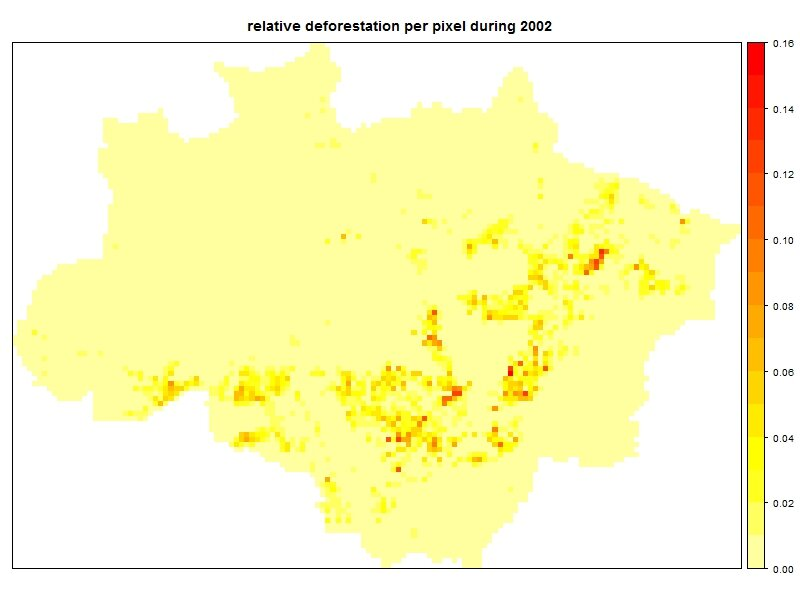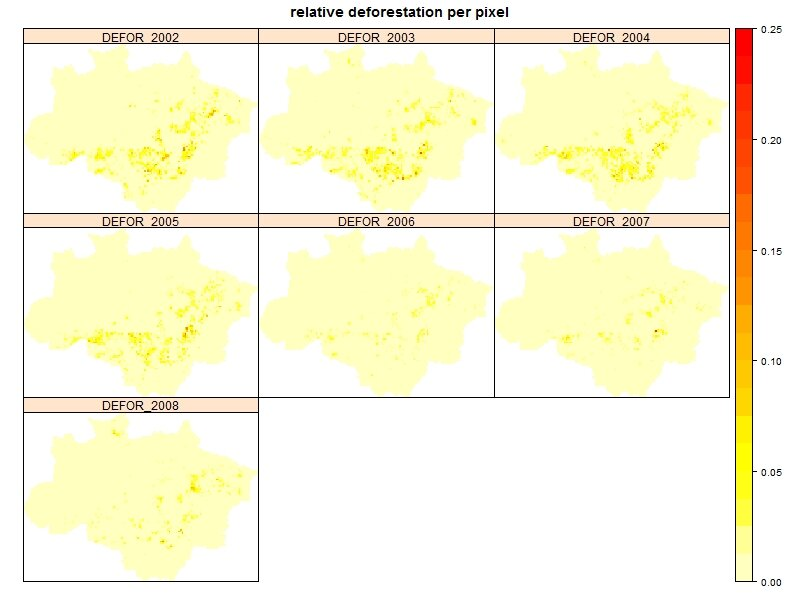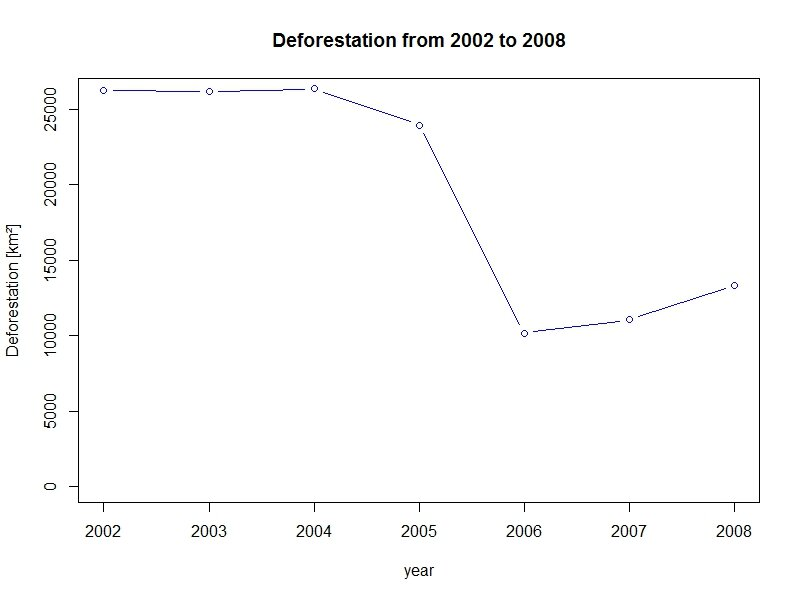# Tutorial part I – Using the SPARQL Package in R to handle Spatial Linked Data

Tools are major enablers of Linked Science. One crucial aspect is how to access and analyse data, and especially how to get only that part of data which is of interest for a given research question.  Linked Data solves the access part, and SPARQL allows to query only a subset of the data. For statistical computing there are tools like R (a open-source software environment for statistical computing).

For analysing processes and operations of complex systems such as environmental and societal systems there is a need to have 1) well interconnected data about a system and 2) techniques for statistical computing and for other types of reasoning to find new information, and means to 3) explore and visualize this information.

As a solution to bridge the two communities, those of statistical computing and  semantic web, there is now the SPARQL Package for R, which enables to get data from Linked Data services to R for analysis. Spatial data in R is widely represented through objects provided by the package sp – as the  following tutorial will do as well.

This tutorial shows

1. how to access Linked Spatio-Temporal Data about the complex environmental system of the Brazilian Amazon Rainforest, including deforestation statistics, and a variety of socio-economic variables,
2. how to store the Spatio-Temporal data in a meaningful way and
3. how to plot and handle it within R

The complete script is available for download from GitHub: linkedAmazon_1.R.

### Accessing the data

At first, make sure that you have recent versions of the two R packages SPARQL and sp installed. Load the two packages by calling:

```library(SPARQL)
library(sp)```

Define the endpoint that will provide you with the triples by

`endpoint <- "http://spatial.linkedscience.org/sparql"`

To reduce the XML’s file size, the data is queried piece-wise. The query is initiated by

```q <- "SELECT ?cell ?row ?col ?polygon
WHERE {
?cell a <http://linkedscience.org/lsv/ns#Item> ;
<http://observedchange.com/tisc/ns#geometry> ?polygon .
}"

res <- SPARQL(url=endpoint, q)\$results```

and completed within a loop over all deforestation variables

```for(var in c("DEFOR_2002", "DEFOR_2003", "DEFOR_2004", "DEFOR_2005", "DEFOR_2006",
"DEFOR_2007","DEFOR_2008")) {
tmp_q <- paste("SELECT ?cell ?",var,"\n WHERE { \n ?cell a <http://linkedscience.org/lsv/ns#Item> ;\n <http://spatial.linkedscience.org/context/amazon/",var,"> ?",var," .\n }\n",sep="")
cat(tmp_q)
res <- merge(res, SPARQL(endpoint, tmp_q)\$results, by="cell")
}```

### Creating a SpatialPixelsDataFrame

We copy the results to a new object and flip the y-axis:

```amazon <- res
amazon\$row <- -res\$row```

Assigning coordinates to a data.frame will result in a Spatial-object. Setting the type to gridded will produce a SpatialPixelsDataFrame:

```coordinates(amazon) <- ~ col+row
gridded(amazon) <- TRUE```

### Plotting and handling the data

As a first application, we produce a map showing relative deforestation per pixel during 2002 by:

```spplot(amazon,"DEFOR_2002",col.regions=rev(heat.colors(17))[-1], at=(0:16)/100,
main="relative deforestation per pixel during 2002")```The full data can be shown as time series of maps for the years from 2002 to 2008.

```spplot(amazon, c("DEFOR_2002", "DEFOR_2003", "DEFOR_2004", "DEFOR_2005",
"DEFOR_2006", "DEFOR_2007","DEFOR_2008"),
col.regions=rev(heat.colors(26))[-1], at=(0:20)/80,as.table=T,
main="relative deforestation per pixel")```Finally, we calculate the cumulative absolute deforestation per year and plot our findings:

```# assuming grid cells of 25km x 25km
cumDefor <- apply(amazon@data[,-c(1,2)],2,function(x) sum(x)*25*25)

plot(2002:2008,cumDefor,type="b", col="blue", ylab="Deforestation [km²]",
xlab="year", main="Deforestation from 2002 to 2008", ylim=c(0,26000))```If you are done with this part I of the tutorial, then you may want to go to the part II of the SPARQL tutorial.

Credits about the script and the Linked Brazilian Amazon Rainforest Data used in this tutorial.

Linked Brazilian Amazon Rainforest Data:

• Aggregation of the spatiotemporal Brazilian Amazon Rainforest data made by Giovana M. de Espindola .
• Publication of the data using Linked Open Data technologies made
by Dr. Tomi Kauppinen  with the help of Jim Jones and Alber Sanchez .

Script for R to analyze the data:

For citing this tutorial please use the following publications:

 Earth System Science Center, National Institute for Space Research (INPE),
Av dos Astronautas 1758, 12227-010 Sao Jose dos Campos Brazil
 http://kauppinen.net/tomi
Institute for Geoinformatics, University of Muenster,
Weseler Strasse 253, 48151 Muenster, Germany
 Institute for Geoinformatics, University of Muenster,
Weseler Strasse 253, 48151 Muenster, Germany